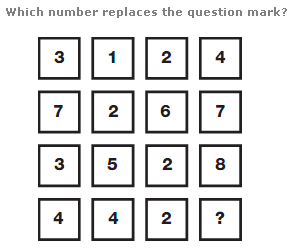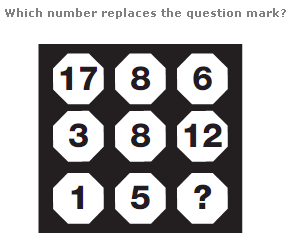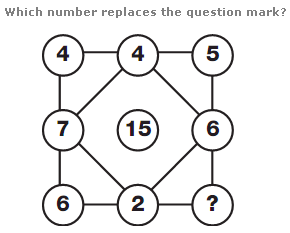# Puzzles - Number puzzles

Exercise : Number puzzles
256.:
0
Explanation
:
Working through the diagram in rows, the right hand value equals the sum of any odd numbers in the rest of the row.

257.:
3
Explanation
:
The sum of the numbers in each column of the diagram is always 21.

258.